# Lecture 15: Drawing General Graphs I

## Announcements

1. Assignment 07 posted, due Friday
2. Assignment 08 posted soon, due next Friday
3. Final Projects
• work with partner
• topic: open-ended
• requirement: build an interactive site with a significant algorithmic component
4. Limited OH This Week (advising week)
• Short OH today
• No OH on Thursday

## Quiz 03, Question 1

Apply matrix(1, 1, -1, 1, 5, 2)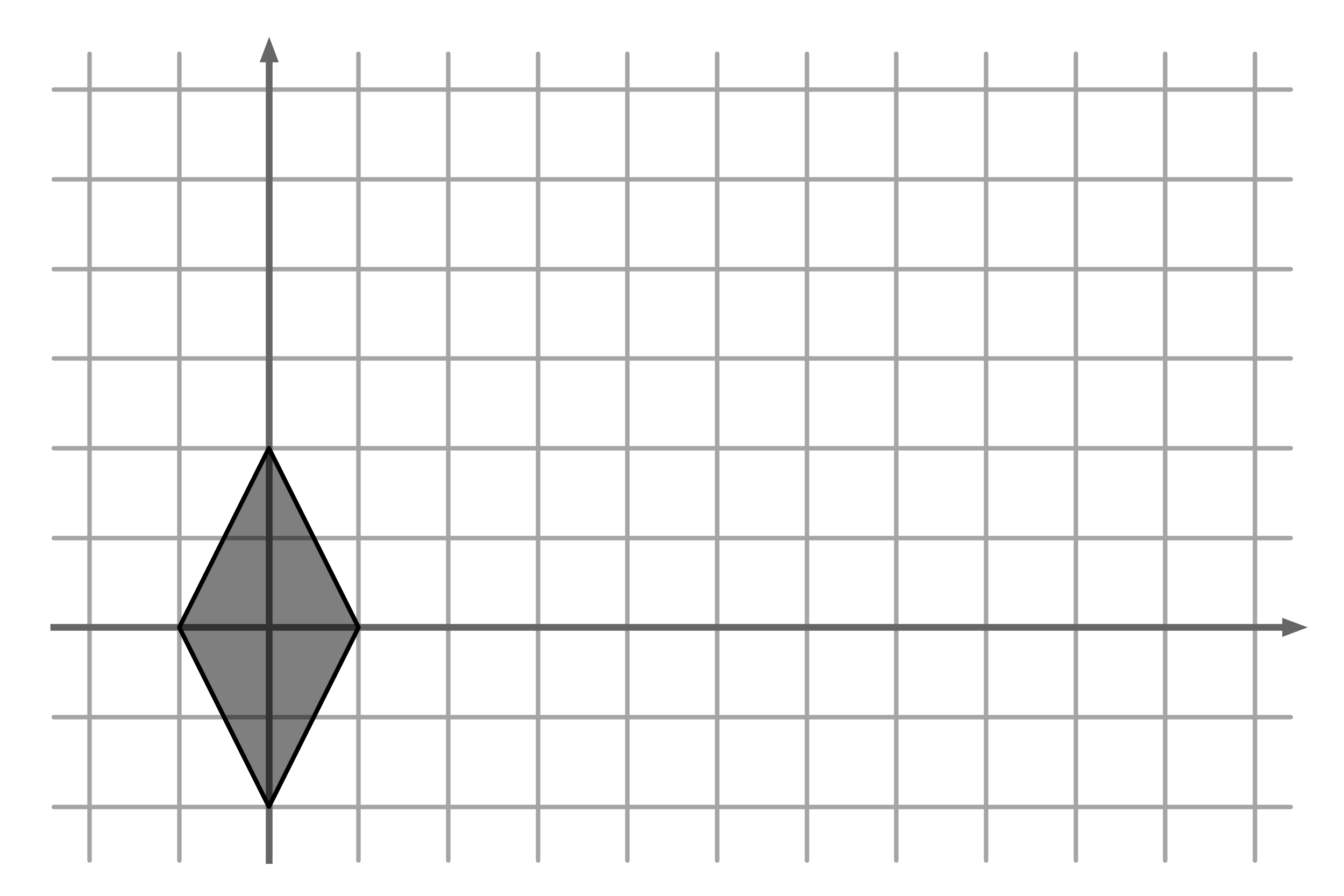## Outline

1. Drawing General Graphs
2. Circular Layouts
• Mäkinen
• AVSDF

## Last Time: Drawing Trees I

Greedy Layout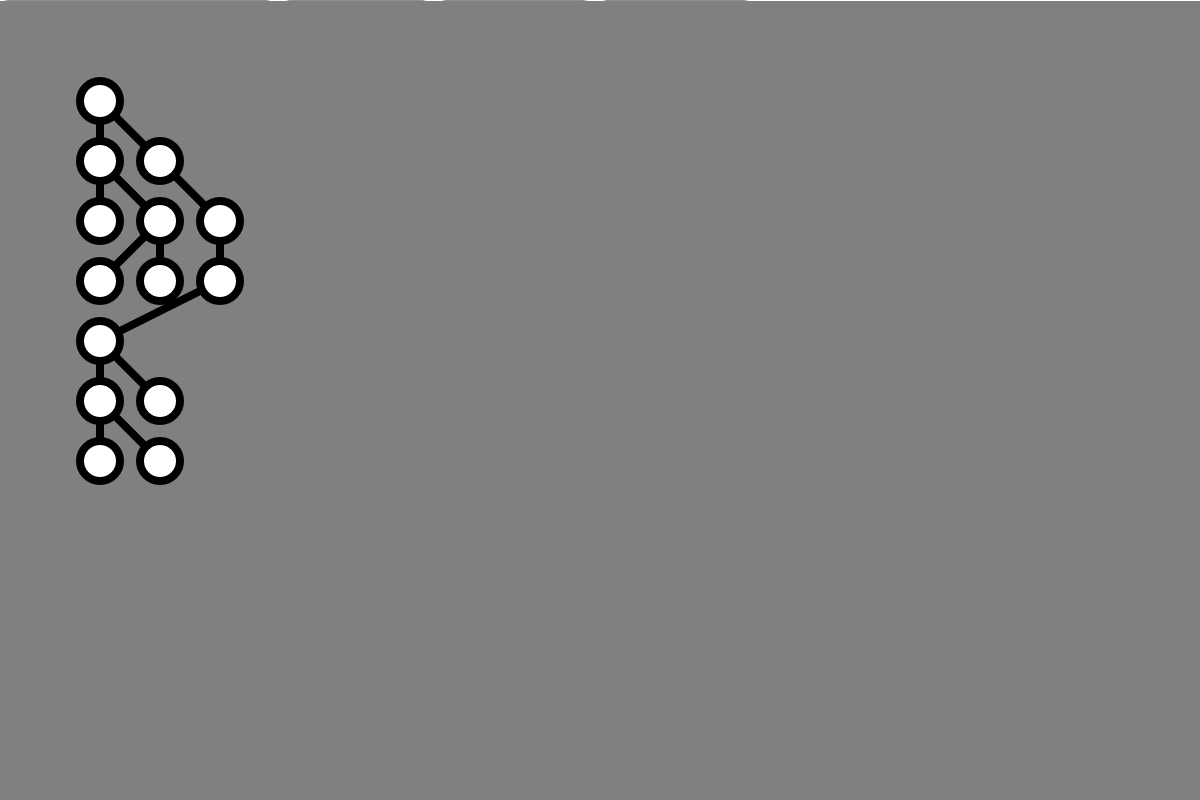## Last Time: Drawing Trees II

Knuth Layout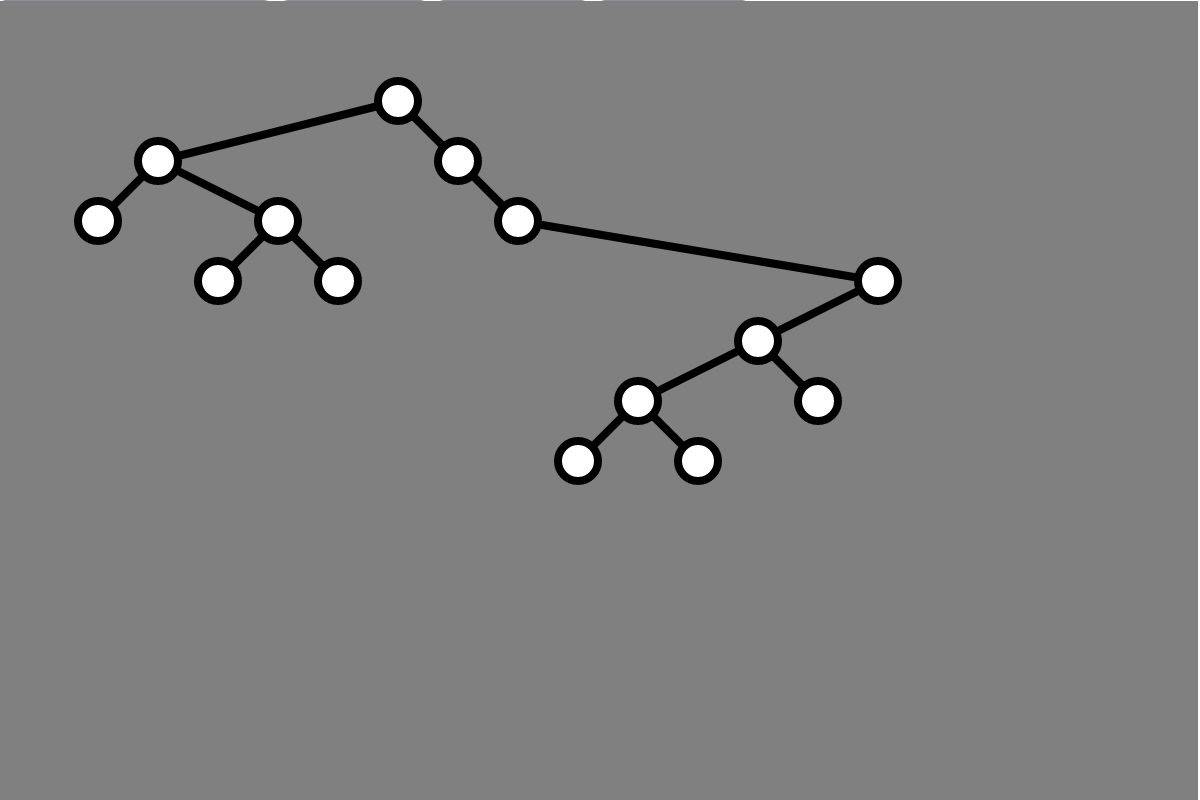## Last Time: Drawing Trees III

Tidy Layout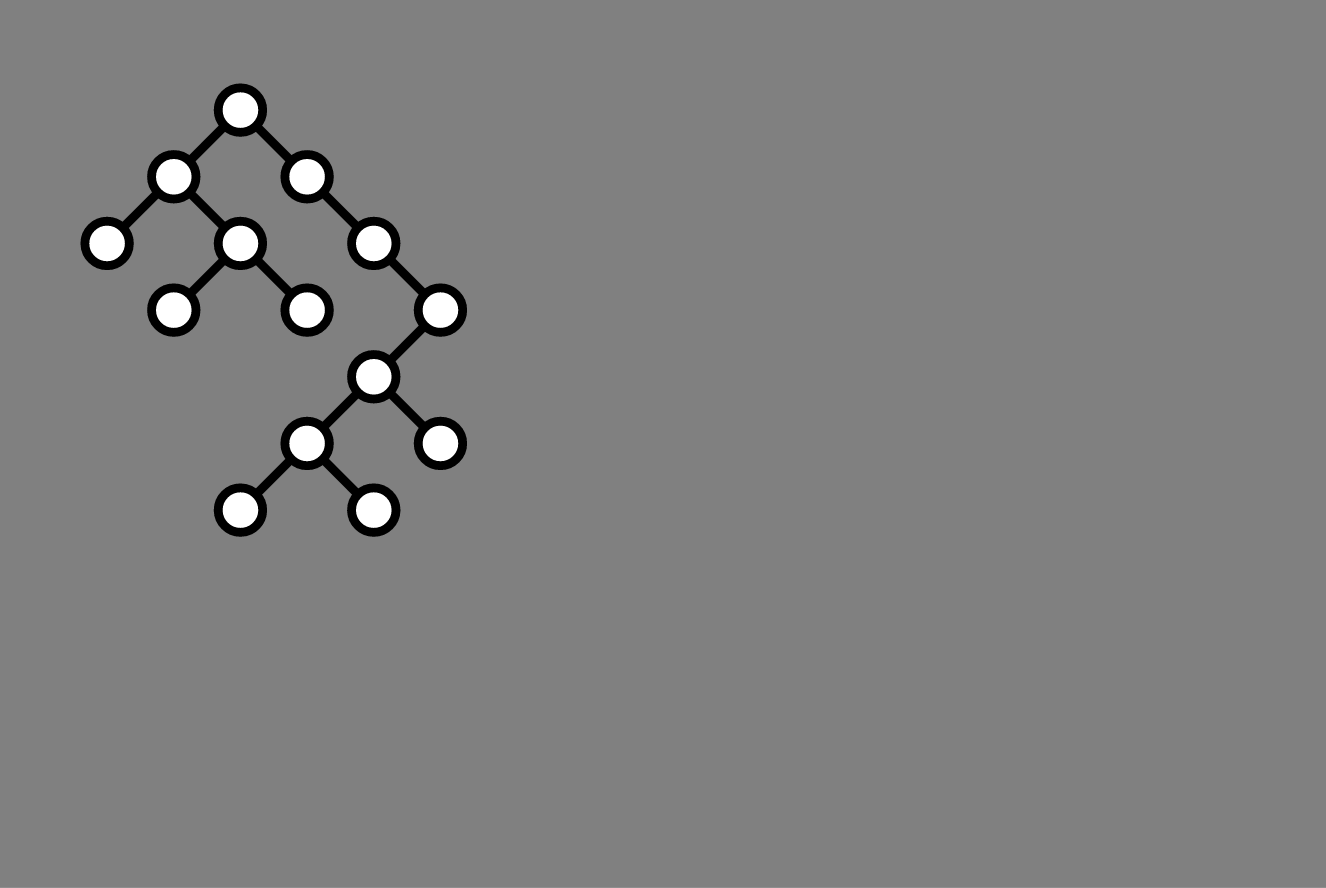## Today

Drawing general graphs

## Warmup Activity

Draw a graph with the following adjacency lists

1: 6, 4, 7
2: 8, 5, 3
3: 6, 2, 4
4: 5, 3, 1
5: 4, 2, 7
6: 1, 3, 8
7: 5, 8, 1
8: 2, 6, 7


## Random Layout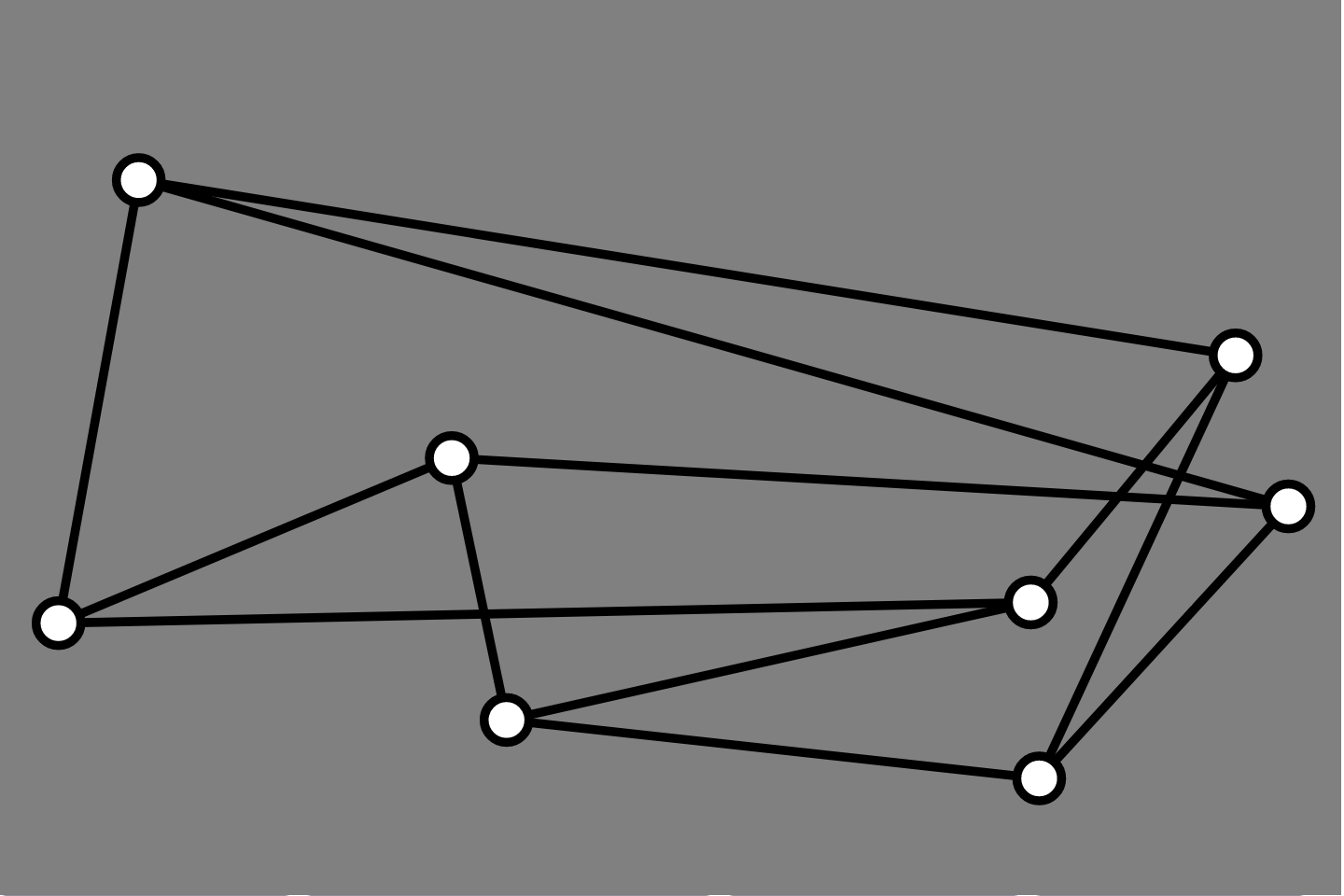## More Generally

What might we want in a graph layout?

## Desiderata

From Fruchterman and Reingold (1991):

1. Distribute the vertices evenly in the frame.
2. Minimize edge crossings.
3. Make edge lengths uniform.
4. Reflect inherent symmetry.
5. Conform to the frame.

## Interesting Question

Which graphs can be drawn without any edge crossings?

• such graphs are called planar graphs

## Algorithmic Results

There are efficient algorithms for

1. detecting if a graph is planar
2. drawing a planar graph without edge crossings, e.g.:
• Auslander-Parter 1961
• Lempel-Even-Cederbaum 1967

Implementing one would make an awesome final project!

## Minimizing Edge Crossings

What about non-planar graphs? (Most graphs are not planar!)

Question. Can we efficiently draw graphs so as to minimize the number of edge crossings?

• there is no known efficient algorithm for this task

More precisely. The following problem is NP-complete

• Input: A graph $G$ and a natural number $k$
• Output: “yes” if $G$ can be drawn with at most $k$ crossings, and “no” otherwise

## General Graph Drawing

Focus on heuristics

• do not guarantee that output minimized edge crossings, etc
• nonetheless have reasonably good results for the graphs we care about
• typically “simple” procedures

## Two (of many) Approaches

1. Circular Layouts (today)
• fix vertices lie on a circle
• pick an ordering of vertices to illustrate some graph features
2. Physical simulation layouts (Wednesday)
• force-directed graphs
• adjacent vertices attract each other (somewhat)
• non-adjacent vertices repel each other
• simulate physical system to determine vertex placement

# Circular Layouts

## Progression I: Random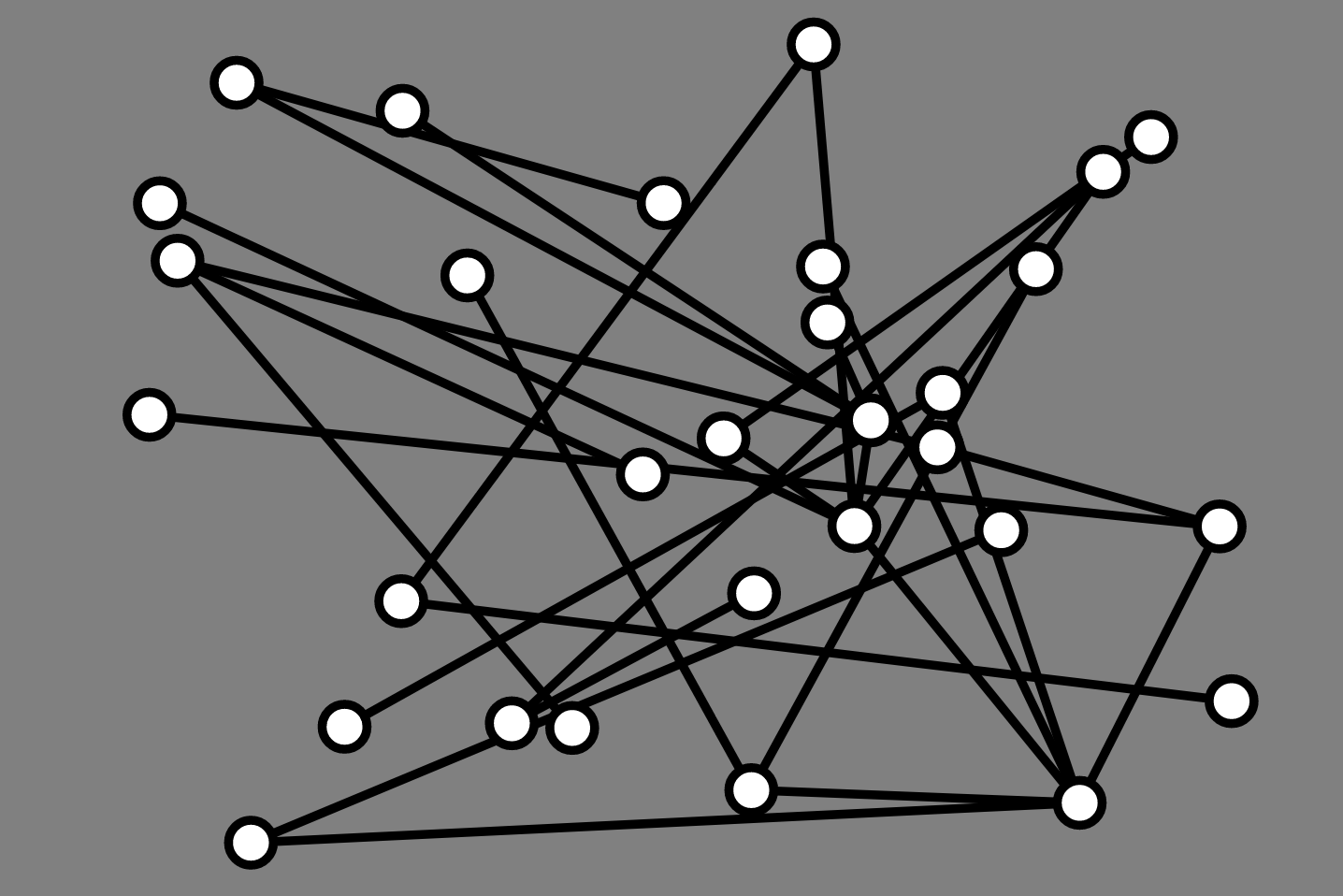## Progression II: Circular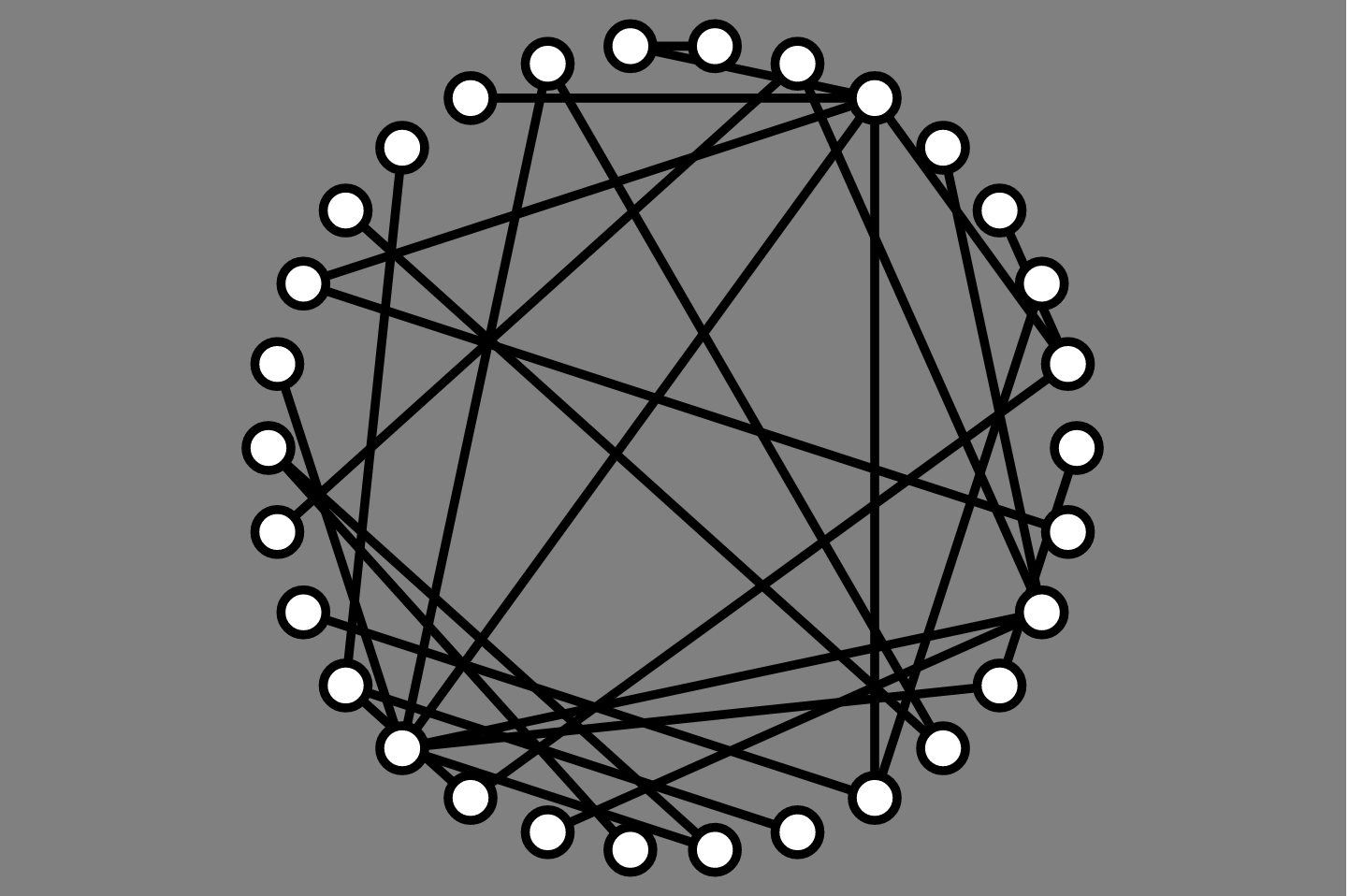## Progression III: AVSDF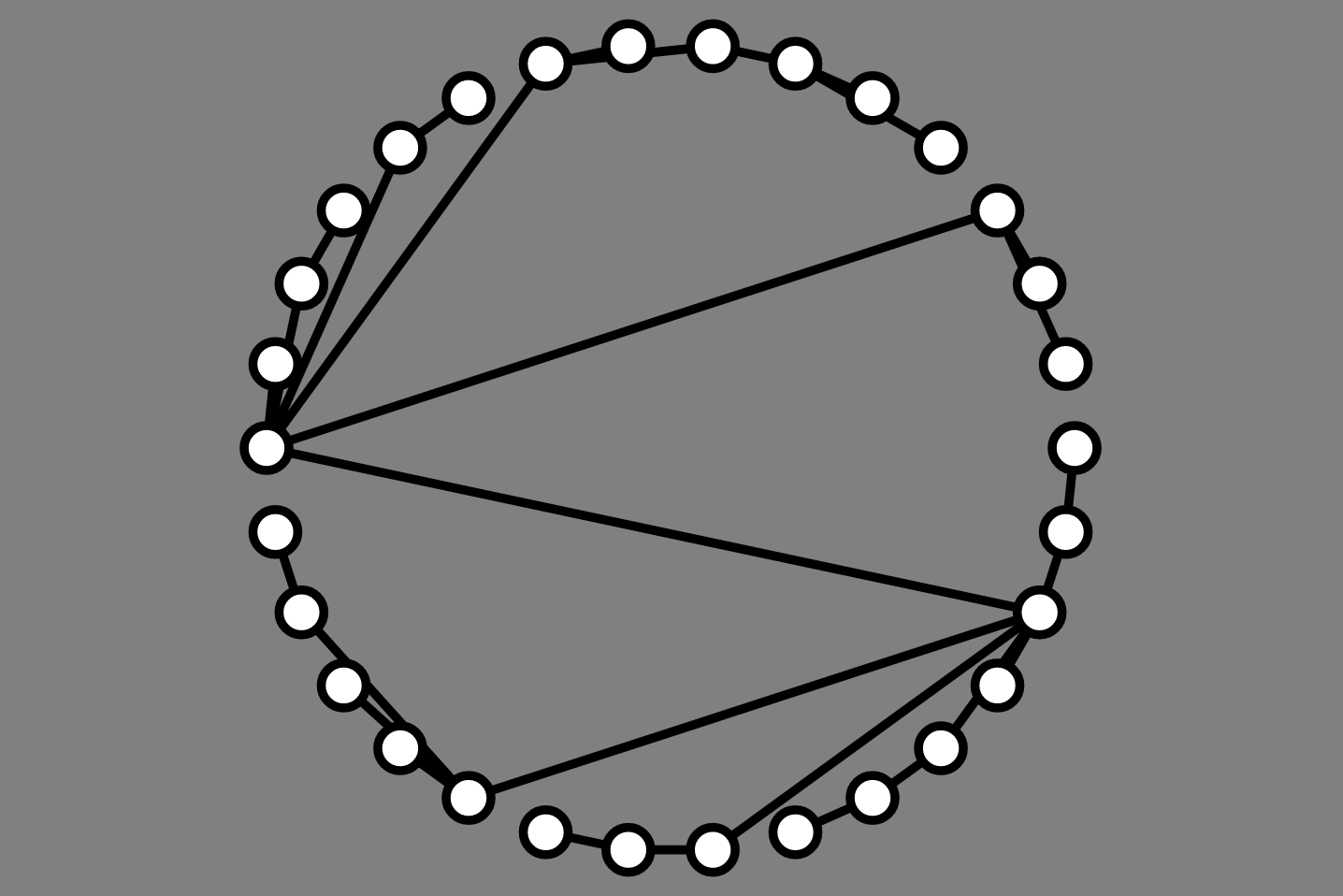## Starting Point

Framework from graph/DFS demos

• Graph object stores lists of vertices/edges
• Vertex object stores adjacency list (neighbors), has x, y
• Edge object represents a pair of vertices
• GraphVisualizer moderates interactions between site and Graph instance
• draws vertices/edges
• responds to user interactions

Previously:

• click pair of vertices to add edge

• hover to highlight a vertex and its neighbors
• demo: lec15-graph-drawing.zip

## Implementing Hover Interactions

Added event listener to each vertex element

	elt.addEventListener("mouseover", (e) => {
this.muteAll();
this.unmuteVertex(vtx);
this.highlightVertex(vtx);
for (let nbr of vtx.neighbors) {
this.highlightVertex(nbr);
this.highlightEdge(this.graph.getEdge(vtx, nbr));
}
});

	elt.addEventListener("mouseout", (e) => {
this.unmuteAll();
this.unhighlightAll();
});


## Circular Embeddings

Setup: Graph with $n$ vertices. How to set locations on a circle?

## Circular Embedding in Code

this.setLayoutCircle = function (cx, cy, r) {
let vertices = this.graph.vertices;
let n = vertices.length;
for (let i = 0; i < n; i++) {
vertices[i].x = r * Math.cos(2 * Math.PI * i / n) + cx;
vertices[i].y = r * Math.sin(2 * Math.PI * i / n) + cy;
}}


## Simplified Problem

Now that we can draw vertices evenly around a circle, we can focus on the order in which to add vertices

• which ordering minimizes edge crossings?
• no easier than general problem!
• which ordering is most informative?
• which ordering looks nice?

## Mäkinen Heuristic

Basic idea:

• split vertices into left and right sets
• vertices with more left neighbors placed on left side
• sim for right side

## Mäkinen Procedure

1. Find two vertices of highest degree and add them to left/right sets
2. Repeat until all vertices are added to left or right:
• compute (right neighbors) - (left neighbors) for each vertex
• add vertex with largest value to right
• add vertex with smallest value to left
3. Add left vertices on left side, right on right side

## Mäkinen Example

1: 2, 6, 3, 5
2: 1, 3, 5, 6
3: 1, 2, 6
4: 2, 5
5: 1, 2, 4
6: 1, 3


## How To Implement Mäkinen Efficiently

• What do we keep track of and store?
• How do we update data structures?
• How efficient is the procedure

## Mäkinen Procedure, Again

1. Find two vertices of highest degree and add them to left/right sets
2. Repeat until all vertices are added to left or right:
• compute (right neighbors) - (left neighbors) for each vertex
• add vertex with largest value to right
• add vertex with smallest value to left
3. Add left vertices on left side, right on right side

## Data Structures

	const vertices = this.graph.vertices;
const n = vertices.length;
const leftPlaced = [];
const rightPlaced = [];
const placed = new Array(n).fill(false);
const leftCount = new Array(n).fill(0);
const rightCount = new Array(n).fill(0);
let placedCount = 2;


## Initialization

	vertices.sort((u, v) => {
return u.degree() - v.degree();
});

// two highest degree vertices go on left and right sides
let left = vertices[n-1];
leftPlaced.push(left);
placed[left.id] = true;
let right = vertices[n-2];
rightPlaced.push(right);
placed[right.id] = true;


## Main Loop I

for (let vtx of left.neighbors) { leftCount[vtx.id]++; }
for (let vtx of right.neighbors) { rightCount[vtx.id]++; }

for (let vtx of vertices) {
if (!placed[vtx.id]) {
left = vtx;
right = vtx;
break;
}}


## Main Loop II

// set right and left to be the vertices maximizing and
// minimizing (respectively) the quantity rightCount -
// leftCount
for (let vtx of vertices) {
if (/* most right - left nbrs */) {
right = vtx;
}

if (/* least right - left nbrs  */) {
left = vtx;
}}


## What is Overall Running Time?

Assume graph has $n$ vertices, $m$

## AVSDF Heuristic

• He & Sykora

Idea:

• perform depth-first search, starting from vertex of minimal degree
• always explore minimum degree neighbor first

## AVSDF Example

1: 2, 6, 3, 5
2: 1, 3, 5, 6
3: 1, 2, 6
4: 2, 5
5: 1, 2, 4
6: 1, 3


## How To Implement AVSDF Efficiently

• What do we keep track of and store?
• How do we update data structures?
• How efficient is the procedure

## AVSDF Initialization

	const order = [];
const stack = [];
const vertices = this.graph.vertices;
const n = vertices.length;
const placed = new Array(n).fill(false);

vertices.sort((u, v) => {
return u.degree() - v.degree();
});

stack.push(vertices);


## Main Loop

while (stack.length > 0) {
let vtx = stack.pop();
if (!placed[vtx.id]) {
order.push(vtx);
placed[vtx.id] = true;
vtx.neighbors.sort((u, v) => {
return v.degree() - u.degree();
});
for (let nbr of vtx.neighbors) {
if (!placed[nbr.id]) { stack.push(nbr); }
}}}


## Next Time

Force-directed layout